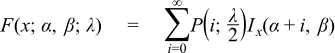#Boost C++ Libraries

...one of the most highly regarded and expertly designed C++ library projects in the world.

This is the documentation for an old version of boost. Click here for the latest Boost documentation.
##### Noncentral Beta Distribution

`#include <boost/math/distributions/non_central_beta.hpp>`

```namespace boost{ namespace math{

template <class RealType = double,
class Policy   = policies::policy<> >
class non_central_beta_distribution;

typedef non_central_beta_distribution<> non_central_beta;

template <class RealType, class Policy>
class non_central_beta_distribution
{
public:
typedef RealType  value_type;
typedef Policy    policy_type;

// Constructor:
non_central_beta_distribution(RealType alpha, RealType beta, RealType lambda);

// Accessor to shape parameters:
RealType alpha()const;
RealType beta()const;

// Accessor to non-centrality parameter lambda:
RealType non_centrality()const;
};

}} // namespaces
```

The noncentral beta distribution is a generalization of the Beta Distribution.

It is defined as the ratio X = χm2(λ) / (χm2(λ) + χn2) where χm2(λ) is a noncentral χ2 random variable with m degrees of freedom, and χn2 is a central χ2 random variable with n degrees of freedom.

This gives a PDF that can be expressed as a Poisson mixture of beta distribution PDFs:where P(i;λ/2) is the discrete Poisson probablity at i, with mean λ/2, and Ix'(α, β) is the derivative of the incomplete beta function. This leads to the usual form of the CDF as:The following graph illustrates how the distribution changes for different values of λ:##### Member Functions
```non_central_beta_distribution(RealType a, RealType b, RealType lambda);
```

Constructs a noncentral beta distribution with shape parameters a and b and non-centrality parameter lambda.

Requires a > 0, b > 0 and lambda >= 0, otherwise calls domain_error.

```RealType alpha()const;
```

Returns the parameter a from which this object was constructed.

```RealType beta()const;
```

Returns the parameter b from which this object was constructed.

```RealType non_centrality()const;
```

Returns the parameter lambda from which this object was constructed.

##### Non-member Accessors

However, the following are not currently implemented: mean, variance, standard deviation, skewness, kurtosis and kurtosis_excess.

The domain of the random variable is [0, 1].

##### Accuracy

The following table shows the peak errors (in units of epsilon) found on various platforms with various floating point types. No comparison to the R-2.5.1 Math library, or to the FORTRAN implementations of AS226 or AS310 are given since these appear to only guarantee absolute error: this would causes our test harness to assign an "infinite" error to these libraries for some of our test values when measuring relative error. Unless otherwise specified any floating-point type that is narrower than the one shown will have effectively zero error.

Table 13. Errors In CDF of the Noncentral Beta

Significand Size

Platform and Compiler

α, β,λ < 200

α,β,λ > 200

53

Win32, Visual C++ 8

Peak=620 Mean=22

Peak=8670 Mean=1040

64

RedHat Linux IA32, gcc-4.1.1

Peak=825 Mean=50

Peak=2.5x104 Mean=4000

64

Redhat Linux IA64, gcc-3.4.4

Peak=825 Mean=30

Peak=1.7x104 Mean=2500

113

HPUX IA64, aCC A.06.06

Peak=420 Mean=50

Peak=9200 Mean=1200

Error rates for the PDF, the complement of the CDF and for the quantile functions are broadly similar.

##### Tests

There are two sets of test data used to verify this implementation: firstly we can compare with a few sample values generated by the R library. Secondly, we have tables of test data, computed with this implementation and using interval arithmetic - this data should be accurate to at least 50 decimal digits - and is the used for our accuracy tests.

##### Implementation

The CDF and its complement are evaluated as follows:

First we determine which of the two values (the CDF or its complement) is likely to be the smaller, the crossover point is taken to be the mean of the distribution: for this we use the approximation due to: R. Chattamvelli and R. Shanmugam, "Algorithm AS 310: Computing the Non-Central Beta Distribution Function", Applied Statistics, Vol. 46, No. 1. (1997), pp. 146-156.Then either the CDF or its complement is computed using the relations:The summation is performed by starting at i = λ/2, and then recursing in both directions, using the usual recurrence relations for the Poisson PDF and incomplete beta functions. This is the "Method 2" described by:

Denise Benton and K. Krishnamoorthy, "Computing discrete mixtures of continuous distributions: noncentral chisquare, noncentral t and the distribution of the square of the sample multiple correlation coefficient", Computational Statistics & Data Analysis 43 (2003) 249-267.

Specific applications of the above formulae to the noncentral beta distribution can be found in:

Russell V. Lenth, "Algorithm AS 226: Computing Noncentral Beta Probabilities", Applied Statistics, Vol. 36, No. 2. (1987), pp. 241-244.

H. Frick, "Algorithm AS R84: A Remark on Algorithm AS 226: Computing Non-Central Beta Probabilities", Applied Statistics, Vol. 39, No. 2. (1990), pp. 311-312.

Ming Long Lam, "Remark AS R95: A Remark on Algorithm AS 226: Computing Non-Central Beta Probabilities", Applied Statistics, Vol. 44, No. 4. (1995), pp. 551-552.

Harry O. Posten, "An Effective Algorithm for the Noncentral Beta Distribution Function", The American Statistician, Vol. 47, No. 2. (May, 1993), pp. 129-131.

R. Chattamvelli, "A Note on the Noncentral Beta Distribution Function", The American Statistician, Vol. 49, No. 2. (May, 1995), pp. 231-234.

Of these, the Posten reference provides the most complete overview, and includes the modification starting iteration at λ/2.

The main difference between this implementation and the above references is the direct computation of the complement when most efficient to do so, and the accumulation of the sum to -1 rather than subtracting the result from 1 at the end: this can substantially reduce the number of iterations required when the result is near 1.

The PDF is computed using the methodology of Benton and Krishnamoorthy and the relation:Quantiles are computed using a specially modified version of bracket_and_solve_root, starting the search for the root at the mean of the distribution. (A Cornish-Fisher type expansion was also tried, but while this gets quite close to the root in many cases, when it is wrong it tends to introduce quite pathological behaviour: more investigation in this area is probably warranted).